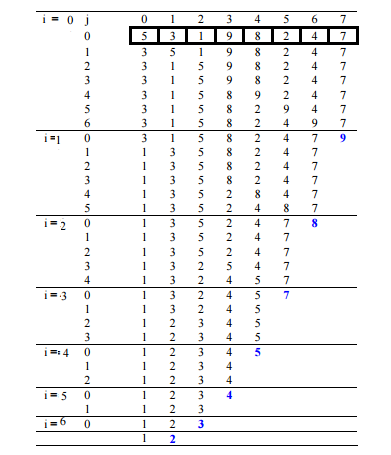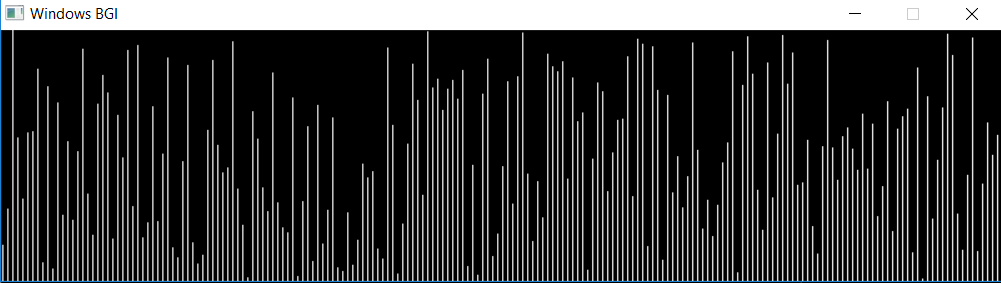# Sorting Algorithms Visualization : Bubble Sort

The human brain can easily process visuals in spite of long codes to understand the algorithms. In this article, Bubble sort visualization has been implemented using graphics.h library. As we all know that bubble sort swaps the adjacent elements if they are unsorted and finally the larger one being shifted towards to the end of array in each pass. Sometimes, it becomes difficult to analyze the data manually, but after plotting graphically it is much easier to understand as shown in the figure below.Approach:

• Even comparing two types of data-set is also difficult with numbers if the size of the array is large.
• The graphical representation of randomly distributed numbers and reverse sorted numbers is shown below.
• The white line is used to represent the length of number (9 being represented by 9 pixels vertically upwards) while its position represents its index in the array.
• Graphically sorting can be shown simply by swapping the lines.
• As we swap the numbers in bubble sort, a different colour line can be used to see the current index in the array (here green colour).
• Here delay() can be increased to see the transition in the graph.

Examples:

```Random arrayReverse sorted array
```

Pre-defined Functions Used:

• setcurrentwindow():A function which is used to set the size of current window.
• setcolor(n): A function which is used to change the color of cursor by changing the value of n.
• delay(n): A function which is used to delay the program by n milliseconds. It is being used for slowing down the transitions speed
• line(x1, y1, x2, y2): A function which is used to draw an line from point (x1, y1) to point (x2, y2). (0, 0) being the left top corner of the screen and bottom right be (n1, n2) where n1, n2 are the width and height of the current window. There are other graphics which can be applied to this line using setcolor().

Below is the program to visualize the Bubble Sort algorithm:

Implementation

 `// C++ program for visualization of bubble sort ` ` `  `#include "graphics.h" ` `#include ` ` `  `using` `namespace` `std; ` ` `  `// Initialize the size ` `// with the total numbers to sorted ` `// and the gap to be maintained in graph ` `vector<``int``> numbers; ` `int` `size = 200; ` `int` `gap = 4; ` ` `  `// Function for swapping the lines graphically ` `void` `swap(``int` `i, ``int` `j, ``int` `x, ``int` `y) ` `{ ` `    ``// Swapping the first line with the correct line ` `    ``// by making it black again and then draw the pixel ` `    ``// for white color. ` ` `  `    ``setcolor(GREEN); ` `    ``line(i, size, i, size - x); ` `    ``setcolor(BLACK); ` `    ``line(i, size, i, size - x);  ` `    ``setcolor(WHITE); ` `    ``line(i, size, i, size - y);  ` ` `  `    ``// Swapping the first line with the correct line ` `    ``// by making it black again and then draw the pixel ` `    ``// for white color. ` `    ``setcolor(GREEN); ` `    ``line(j, size, j, size - y); ` `    ``setcolor(BLACK); ` `    ``line(j, size, j, size - y); ` `    ``setcolor(WHITE); ` `    ``line(j, size, j, size - x); ` `} ` ` `  `// Bubble sort function ` `void` `bubbleSort() ` `{ ` `    ``int` `temp, i, j; ` ` `  `    ``for` `(i = 1; i < size; i++) { ` `        ``for` `(j = 0; j < size - i; j++) { ` `            ``if` `(numbers[j] > numbers[j + 1]) { ` `                ``temp = numbers[j]; ` `                ``numbers[j] = numbers[j + 1]; ` `                ``numbers[j + 1] = temp; ` ` `  `                ``// As we swapped the last two numbers ` `                ``// just swap the lines with the values. ` `                ``// This is function call ` `                ``// for swapping the lines ` `                ``swap(gap * j + 1, ` `                     ``gap * (j + 1) + 1, ` `                     ``numbers[j + 1], ` `                     ``numbers[j]); ` `            ``} ` `        ``} ` `    ``} ` `} ` ` `  `// Driver program ` `int` `main() ` `{ ` ` `  `    ``// auto detection of screen size ` `    ``int` `gd = DETECT, gm; ` `    ``int` `wid1; ` ` `  `    ``// Graph initialization ` `    ``initgraph(&gd, &gm, NULL); ` ` `  `    ``// setting up window size (gap*size) * (size) ` `    ``wid1 = initwindow(gap * size + 1, size + 1); ` `    ``setcurrentwindow(wid1); ` ` `  `    ``// Initializing the array ` `    ``for` `(``int` `i = 1; i <= size; i++) ` `        ``numbers.push_back(i); ` ` `  `    ``// Find a seed and shuffle the array ` `    ``// to make it random. ` `    ``// Here  different type of array ` `    ``// can be taken to results ` `    ``// such as nearly sorted, already sorted, ` `    ``// reverse sorted to visualize the result ` `    ``unsigned seed ` `        ``= chrono::system_clock::now() ` `              ``.time_since_epoch() ` `              ``.count(); ` ` `  `    ``shuffle(numbers.begin(), ` `            ``numbers.end(), ` `            ``default_random_engine(seed)); ` ` `  `    ``// Initial plot of numbers in graph taking ` `    ``// the vector position as x-axis and its ` `    ``// corresponding value will be the height of line. ` `    ``for` `(``int` `i = 1; i <= gap * size; i += gap) { ` `        ``line(i, size, i, (size - numbers[i / gap])); ` `    ``} ` ` `  `    ``// Delay the code ` `    ``delay(200); ` ` `  `    ``// Call sort ` `    ``bubbleSort(); ` ` `  `    ``for` `(``int` `i = 0; i < size; i++) { ` `        ``cout << numbers[i] << ``" "``; ` `    ``} ` `    ``cout << endl; ` ` `  `    ``// Wait for sometime . ` `    ``delay(5000); ` ` `  `    ``// Close the graph ` `    ``closegraph(); ` ` `  `    ``return` `0; ` `} `

Output:

```1 2 3 4 5 6 7 8 9 10 11 12 13 14 15 16 17 18 19 20 21 22 23 24 25 26 27 28 29 30 31 32
33 34 35 36 37 38 39 40 41 42 43 44 45 46 47 48 49 50 51 52 53 54 55 56 57 58 59 60 61
62 63 64 65 66 67 68 69 70 71 72 73 74 75 76 77 78 79 80 81 82 83 84 85 86 87 88 89 90
91 92 93 94 95 96 97 98 99 100 101 102 103 104 105 106 107 108 109 110 111 112 113 114
115 116 117 118 119 120 121 122 123 124 125 126 127 128 129 130 131 132 133 134 135 136
137 138 139 140 141 142 143 144 145 146 147 148 149 150 151 152 153 154 155 156 157 158
159 160 161 162 163 164 165 166 167 168 169 170 171 172 173 174 175 176 177 178 179 180
181 182 183 184 185 186 187 188 189 190 191 192 193 194 195 196 197 198 199 200

```

Attention reader! Don’t stop learning now. Get hold of all the important DSA concepts with the DSA Self Paced Course at a student-friendly price and become industry ready.

My Personal Notes arrow_drop_upCheck out this Author's contributed articles.

If you like GeeksforGeeks and would like to contribute, you can also write an article using contribute.geeksforgeeks.org or mail your article to contribute@geeksforgeeks.org. See your article appearing on the GeeksforGeeks main page and help other Geeks.

Please Improve this article if you find anything incorrect by clicking on the "Improve Article" button below.

Improved By : Harshit-Agarwal